BetMGM Big 12 Odds
-175
0.57 to 1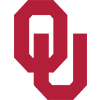Oklahoma
51.0% implied probability

+275
2.75 to 1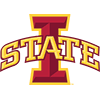Iowa State
21.4% implied probability

+900
9 to 1Texas
8.0% implied probability

+1200
12 to 1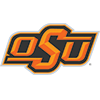Oklahoma State
6.2% implied probability

+1400
14 to 1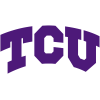TCU
5.3% implied probability

+2500
25 to 1West Virginia
3.1% implied probability

+4000
40 to 1Kansas State
2.0% implied probability

+5000
50 to 1Baylor
1.6% implied probability

No changes have been recorded yet.
+6500
65 to 1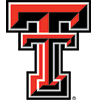Texas Tech
1.2% implied probability

No changes have been recorded yet.
+25000
250 to 1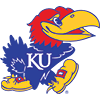Kansas
0.3% implied probability